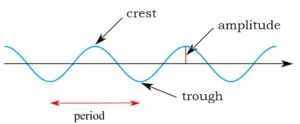Home » frequency

# frequency

« Back to Glossary Index

Frequency is the number of repeated motions in a period of time. These motions can be up and down, like a hammer, or back and forth, like waving a hand. Or some other repeated motion, maybe rotating in a circle. Repeated motions are called “oscillations” or “cycles.” So, frequency is the number of cycles per unit of time. The unit used for time is often seconds.Key wave properties: period, frequency (number of crests per second), and amplitude. The horizontal axis is time. [Image source: modification of Kraaiennest – CC BY-SA 3.0, https://commons.wikimedia.org/w/index.php?curid=4038226. Retrieved Sept. 21, 2017.]

Cycles can be graphed on paper, as in the accompanying image. In the case of the up and down motion of the hammer, the vertical axis would be elevation and the horizontal axis would be time. When the hammer is highest in elevation, it’s a crest. When lowest in elevation, it’s a trough. Then, over time, it rises again in elevation until it’s a crest, and so on.

In this image, frequency is the number of cycles completed in a second. The more cycles per second, the higher the frequency.

Frequency is symbolized by either the ancient Greek letter v (pronounced nu) or is symbolized simply by f for frequency. The symbol v is generally used for the frequency of electromagnetic waves; whereas, the symbol f is generally used for the frequency of ordinary waves that require a material medium, such as sound and water waves.

The time lapse between crests (or between troughs) is called the “period” of the wave. The higher the frequency, the shorter the period. The lower the frequency, the longer the period.

The wavelength is the distance between repeats of the wave, that is, the distance in space between one crest (or one trough) and the next.

See the entry for frequency and wavelength for examples and illustrations as well as the relationship of frequency to:

• Wavelength,
• Wave speed or velocity, and
• Wave energy

This site uses Akismet to reduce spam. Learn how your comment data is processed.